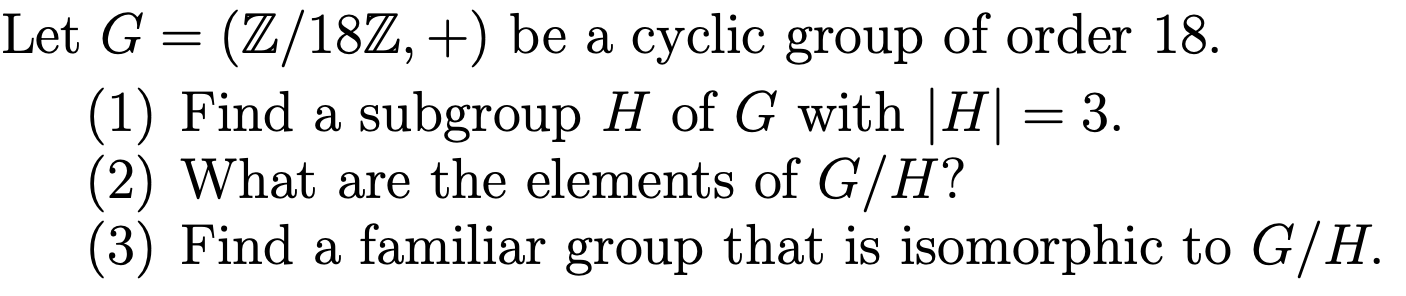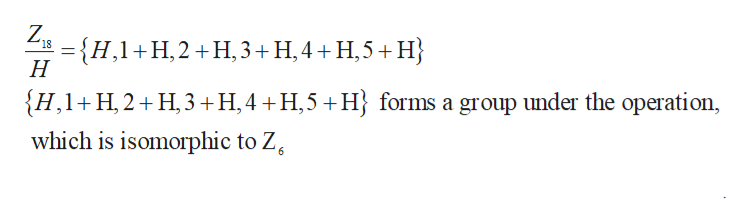# Let G(Z/18Z, +) be a cyclic group of order 18.(1) Find a subgroup H of G with |H= 3(2) What are the elements of G/H?(3) Find a familiar group that is isomorphic to G/H.

Questionhelp_outlineImage TranscriptioncloseLet G (Z/18Z, +) be a cyclic group of order 18. (1) Find a subgroup H of G with |H= 3 (2) What are the elements of G/H? (3) Find a familiar group that is isomorphic to G/H. fullscreen
check_circleExpert Solution
Step 1

The group G = (Z/18Z, +) be a cyclic group of order 18 so, the group G is similar to Z18.

Part (a):

A subgroup H of the group G such that |H|=3. Here 18 is divided by 3.

So, the subgroup of order 3 in a cyclic group of order 18 (Z18) is only one group which is Z3.

And similarly H can be written as:

Step 2

Part (b):

The elements of G/H:help_outlineImage TranscriptioncloseZ, H,1H,2H,3+ H,4+H,5+H Н {H,1 H, 2 H3 H,4 H,5H} forms a group under the operation, which is isomorphic to Z 6 fullscreen
Step 3

Z/H = {(0,6,12),(1,7,13),(2,8,14),(3,9,15),(4,10,16),(5,11,17)}

H={0,6,12}

H+1 = {1,7,13}

H+2 ={2,8,14}

&n...

### Want to see the full answer?

See Solution

#### Want to see this answer and more?

Solutions are written by subject experts who are available 24/7. Questions are typically answered within 1 hour*

See Solution
*Response times may vary by subject and question
Tagged in

### Math